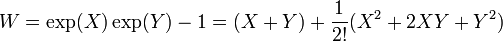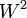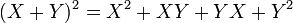# Baker-Campbell-Hausdorff formula for nilpotency class two

## Statement

The Baker-Campbell-Hausdorff formula for nilpotency class two is a special case of the Baker-Campbell-Hausdorff formula that works in the case of nilpotency class two. In particular, it is a formula that can be used to go back and forth along the Baer correspondence.

The explicit formula for$\log(\exp(X)\exp(Y))$ is given in a number of forms below:

Form Expression Primes required to be invertible
sum of associative products$X + Y + \frac{1}{2}(XY) - \frac{1}{2}(YX)$ 2
sum of Lie products (left-normed or right-normed, it doesn't matter)$X + Y + \frac{1}{2}[X,Y]$ 2
sum of basic Lie products (using Khukhro's left-normed convention, treating$Y > X$)$X + Y - \frac{1}{2}[Y,X]$ 2

## Deducing the formula

### Using the explicit formula

The explicit formula says the following. The iterated Lie bracket in this version of the formula is right-normed, i.e.,$[X_1,X_2,X_3]$ stands for$[X_1,[X_2,X_3]]$::$\log(\exp(X)\exp(Y) = \sum_{n>0}\frac {(-1)^{n-1}}{n} \sum_{ \begin{smallmatrix} {r_i + s_i > 0} \\ {1\le i \le n} \end{smallmatrix}} \frac{(\sum_{i=1}^n (r_i+s_i))^{-1}}{r_1!s_1!\cdots r_n!s_n!} [ X^{r_1} Y^{s_1} X^{r_2} Y^{s_2} \ldots X^{r_n} Y^{s_n} ]$

We calculate each of the terms individually for the case$n = 1$. Note that in this case, the expression for the outer sum is$(-1)^{1-1}/1 = 1$:$r_1$$s_1$$r_1 + s_1$$\frac{(\sum_{i=1}^n (r_i+s_i))^{-1}}{r_1!s_1!\cdots r_n!s_n!} [ X^{r_1} Y^{s_1} X^{r_2} Y^{s_2} \ldots X^{r_n} Y^{s_n} ]$, which in this case is just$\frac{1}{r_1!s_1!(r_1 + s_1)} [ X^{r_1} Y^{s_1}]$ Simplified form
1 0 1$\frac{1}{1!0!1}X$$X$
0 1 1$\frac{1}{0!1!1}Y$$Y$
1 1 2$\frac{1}{1!1!2}[X,Y]$$\frac{1}{2}[X,Y]$
2 0 2$\frac{1}{2!0!2}[X,X]$ 0
0 2 2$\frac{1}{0!2!2}[Y,Y]$ 0
Total (--) -- -- --$\! X + Y + \frac{1}{2}[X,Y]$

If$r_1 + s_1 > 1$, the degree becomes greater than two, and the term becomes zero if we are in class two.

For$n = 2$, we have:$r_1$$s_1$$r_2$$s_2$$r_1 + s_1 + r_2 + s_2$$\frac{(\sum_{i=1}^n(r_i+s_i))^{-1}}{r_1!s_1!\cdots r_n!s_n!} [ X^{r_1} Y^{s_1} X^{r_2} Y^{s_2} \ldots X^{r_n} Y^{s_n} ]$, which in this case is just$\frac{1}{r_1!s_1!(r_1 + s_1 + r_2 + s_2)} [ X^{r_1} Y^{s_1} X^{r_2}Y^{s_2}]$ Simplified form
1 0 1 0 2$\frac{1}{1!0!1!0!2}[X,X]$ 0
1 0 0 1 2$\frac{1}{1!0!0!1!2}[X,Y]$$\frac{1}{2}[X,Y]$
0 1 1 0 2$\frac{1}{0!1!1!0!2}[Y,X]$$\frac{1}{2}[Y,X]$
0 1 0 1 2$\frac{1}{1!0!1!0!2}[Y,Y]$ 0
Total (--) -- -- -- -- -- 0

Note that all other terms are zero simply by degree considerations.

Combining both, we obtain that:$\log(\exp(X)\exp(Y)) = X + Y + \frac{1}{2}[X,Y]$

### Using the general procedure

We describe here how the formula can be deduced using the general procedure outlined in deducing the Baker-Campbell-Hausdorff formula from associative algebra manipulation.

#### Quick version

By the quick formula, we have:$W = \exp(X)\exp(Y) -1 = (X + Y) + \frac{1}{2!}(X^2 + 2XY + Y^2)$

Further, since we are in class two,$W^3 = 0$, so we get:$\log(1 + W) = W - \frac{W^2}{2} = (X + Y) + \frac{1}{2!}(X^2 + 2XY + Y^2) - \frac{1}{2}((X + Y) + \frac{1}{2!}(X^2 + 2XY + Y^2))^2$

In the second square term, the class two condition means that the only surviving part is$(X + Y)^2$, so we get:$\log(1 + W) = (X + Y) + \frac{1}{2!}(X^2 + 2XY + Y^2) - \frac{1}{2}(X + Y)^2$

Simplify, and get:$(X + Y) + \frac{1}{2}(X^2 + 2XY + Y^2 - X^2 - XY - YX - Y^2)$

This further simplifies to:$(X + Y) + \frac{1}{2}(XY - YX)$

This can be rewritten as:$(X + Y) + \frac{1}{2}[X,Y]$

#### Detailed version

In this case, we work with non-commuting variables$X,Y$ such that$X^3 = X^2Y = XYX = XY^2 = YX^2 = YXY = Y^2X = Y^3 = 0$. Thus:$\! \exp(X) = 1 + X + \frac{X^2}{2}$$\! \exp(Y) = 1 + Y + \frac{Y^2}{2}$

We thus get:$\exp(X)\exp(Y) = \left(1 + X + \frac{X^2}{2}\right)\left(1 + Y + \frac{Y^2}{2}\right) = 1 + X + \frac{X^2}{2} + Y + XY + \frac{X^2Y}{2} + \frac{Y^2}{2} + \frac{XY^2}{2} + \frac{X^2Y^2}{4}$

We drop all products of degree three or more and rearrange the remaining terms to get:$\! \exp(X)\exp(Y) = 1 + X + Y + \frac{X^2}{2} + XY + \frac{Y^2}{2}$

We thus get:$\! W = \exp(X)\exp(Y) - 1 = X + Y + \frac{X^2}{2} + XY + \frac{Y^2}{2}$

Finally, we compute$\log(1 + W)$. We have:$\log(1 + W) = W - \frac{W^2}{2}$

We note that$W^2$ is the same as the square of its linear part because the square of the degree two part, as well as products of the degree two and the linear part, are degree three or more and hence zero. Thus:$\log(1 + W) = X + Y + \frac{X^2}{2} + XY + \frac{Y^2}{2} - (X + Y)^2/2$

Simplifying, we get:$\log(1 + W) = X + Y + \frac{X^2 + 2XY + Y^2 - (X + Y)^2}{2}$

Now note that:$\! (X + Y)^2 = X^2 + XY + YX + Y^2$

Plugging this in, we get:$\log(1 + W) = X + Y + \frac{X^2 + 2XY + Y^2 - (X^2 + XY + YX + Y^2)}{2}$

Simplifying, we get:$\log(1 + W) = X + Y + \frac{XY - YX}{2}$

Rewrite$XY - YX = [X,Y]$ and we get the formula:$\! X + Y + \frac{1}{2}[X,Y]$